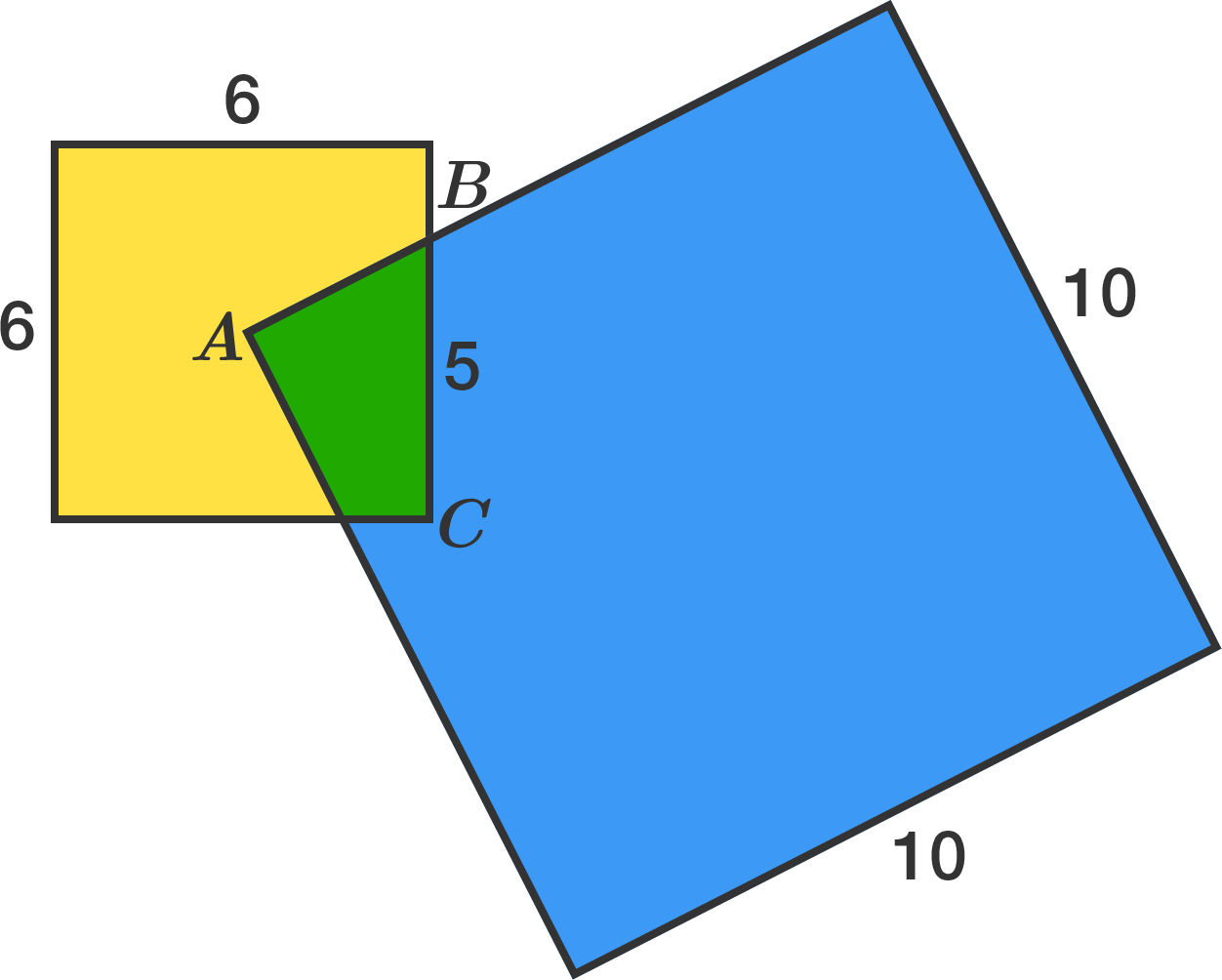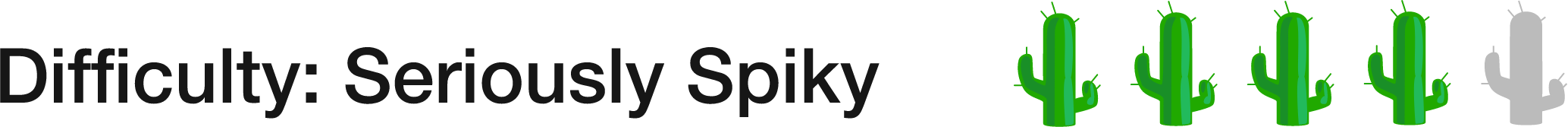# 99 of 100: Penultimate Puzzle

Geometry Level 1A square of side length 10 is placed so that its corner, $A,$ is exactly at the center of a square of side length 6. The squares intersect at point $B$ as shown; the length of line segment $BC$ (connecting $B$ to a corner of the smaller square) is 5.

What is the area of the green quadrilateral?If you get stuck, try drawing some lines on the figure to help you out.

×

Problem Loading...

Note Loading...

Set Loading...